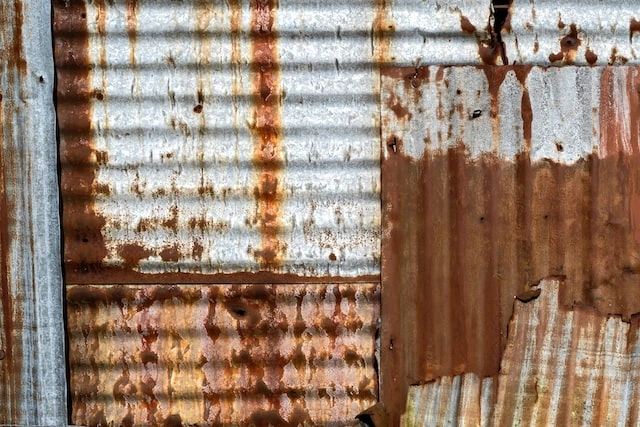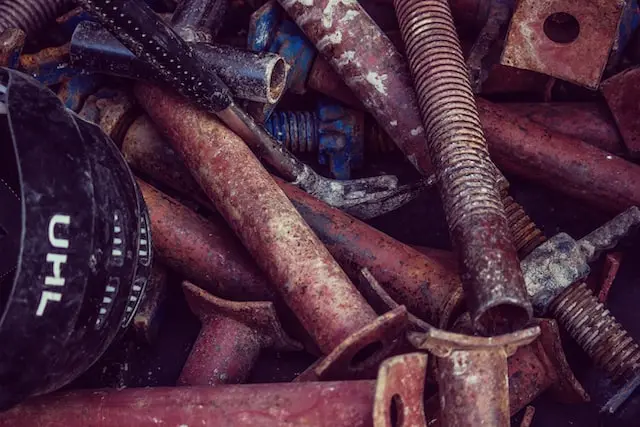Study S3 Chemistry Oxidation and Reduction - Geniebook# Oxidation And Reduction

• Define and identify oxidation and reduction reactions in terms of
• gain or loss of oxygen;
• gain or loss of hydrogen; and
• gain or loss of electrons.

### What do you observe in the images below?Image Credit: Lucas van Oort - unsplash.comImage Credit: Michael Schaffler - unsplash.com

You notice that there is a brown coating on the surfaces of the corrugated iron sheet (on the left) and the nuts and bolts (on the right). The coating is the result of a chemical reaction, involving the process of oxidation and reduction.

## Oxidation vs Reduction

The table below summarises the four different definitions of oxidation and reduction.

 Oxidation Reduction A substance has been oxidised when it: A substance has been reduced when it: gains oxygen. loses oxygen loses hydrogen. gains hydrogen loses electrons. gains electrons increases in its oxidation state. decreases in its oxidation state

When a chemical reaction involves both the oxidation of one substance and the reduction of another substance, it is known as a redox reaction

## Oxidation And Reduction As Gain Or Loss Of Oxygen

A substance is oxidised when it gains oxygen.

A substance is reduced when it loses oxygen.

Example 1:

$$Zn + CuO \;\xrightarrow[\qquad\qquad]{} \; ZnO + Cu$$

Substance oxidised:  Zn is oxidised as Zn has gained oxygen to form ZnO.

Substance reduced: CuO is reduced as CuO has lost oxygen to form Cu.

Example 2:

$$Fe_2O_3 + 3CO \;\xrightarrow[\qquad\qquad]{} \; 2Fe + 3CO_2$$

Substance oxidised: CO is oxidised as CO has gained oxygen to form CO2

Substance reduced: Fe2O3 is reduced as Fe2O3 has lost oxygen to form Fe.

Example 3:

$$Mg + H_2O \;\xrightarrow[\qquad\qquad]{} \; MgO + H_2$$

Substance oxidised: Mg is oxidised as Mg has gained oxygen to form MgO

Substance reduced: H2O is reduced as H2O has lost oxygen to form H2.

Note:

1. When we identify substances undergoing oxidation or reduction, we always refer to the reactants, not the products.
2. It is not necessary to include the coefficient in your answer, i.e. CO instead of 3CO

Question 1:

Study the following chemical reaction. Identify the substance that is reduced, in terms of the gain or loss of oxygen.

$$PbO + CO \;\xrightarrow[\qquad\qquad]{} \; Pb + CO_2$$

1. CO
2. CO2
3. Pb
4. PbO

Solution:

(D) PbO

Explanation:

In the reaction, PbO is reduced as it has lost oxygen to form Pb

Question 2:

Study the following chemical reaction. Identify the substance that is oxidised, in terms of the gain or loss of oxygen.

$$H_2 + CuO \;\xrightarrow[\qquad\qquad]{} \; Cu + H_2O$$

1. Cu
2. CuO
3. H2
4. H2O

Solution:

(C) H2

Explanation:

H2 has gained oxygen to form H2O. Hence, H2 is oxidised.

## Oxidation And Reduction As Gain Or Loss Of Hydrogen

A substance is oxidised when it loses hydrogen.

A substance is reduced when it gains hydrogen.

Example 1:

$$H_2S + Cl_2 \;\xrightarrow[\qquad\qquad]{} \; 2HCl + S$$

Substance oxidised: H2S is oxidised as H2S has lost hydrogen to form S

Substance reduced: Cl2 is reduced as Cl2 has gained hydrogen to form HCl.

Example 2:

$$CH_4 + 2Cl_2 \;\xrightarrow[\qquad\qquad]{} \; C + 4HCl$$

Substance oxidised: CH4 is oxidised as CH4 has lost hydrogen to form C

Substance reduced: Cl2 is reduced as Cl2 has gained hydrogen to form HCl.

Question 3:

Study the following chemical reaction. Identify the substance that is oxidised, in terms of the gain or loss of hydrogen.

$$2NH_3 + 3Br_2 \;\xrightarrow[\qquad\qquad]{} \; N_2 + 6HBr$$

1. Br2
2. HBr
3. N2
4. NH3

Solution:

(D) NH3

Explanation:

NH3 has lost hydrogen to form N2. Hence NH3 is oxidised.

## Oxidation And Reduction As Gain Or Loss Of Electrons

A substance is oxidised when it loses electrons

A substance is reduced when it gains electrons.

Example 1:

$$Na \;\xrightarrow[\qquad\qquad]{} \; Na^+ + e^–$$

Substance oxidised: Na is oxidised as Na has lost an electron to form Na+ ion.

Example 2:

$$Cu \;\xrightarrow[\qquad\qquad]{} \; Cu^{2+} + 2e^–$$

Substance oxidised: Cu is oxidised as Cu has lost 2 electrons to form Cu2+ ion.

Example 3:

$$Na^+ + e^- \;\xrightarrow[\qquad\qquad]{} \; Na$$

Substance reduced: Na+ is reduced as Na+ has gained an electron to form Na.

Example 4:

$$Cu^{2+} + 2e^- \;\xrightarrow[\qquad\qquad]{} \; Cu$$

Substance reduced: Cu2+ is reduced as Cu2+ has gained 2 electrons to form Cu .

Example 5:

$$Cu (s) + 2AgNO_3 (aq) \;\xrightarrow[\qquad\qquad]{} \; Cu(NO_3)_2 (aq) + 2Ag (s)$$

To identify the substance oxidised or reduced, we can construct the ionic equation for this reaction.

$$Cu(s) + 2Ag^+ (aq) \;\xrightarrow[\qquad\qquad]{} \; Cu^{2+} (aq) + 2Ag (s)$$

Substance Oxidised:

Cu is oxidised as Cu has lost 2 electrons to form Cu2+ in Cu(NO3)2

Substance Reduced:

AgNO3 is reduced as Ag+ in AgNO3 has gained an electron to form Ag.

## Try It Yourself

Question 1:

$$Pb(NO_3)_2 (aq) + Mg (s) \;\xrightarrow[\qquad\qquad]{} \; Pb (s) + Mg(NO_3)_2 (aq)$$

1. Write the ionic equation for the reaction shown above.

Solution:

$$Pb^{2+}(aq) + Mg (s) \;\xrightarrow[\qquad\qquad]{} \; Pb (s) + Mg^{2+}(aq)$$

1. Identify the substance that is oxidised. Explain your answer in terms of electron transfer.

Solution:

Mg is oxidised as Mg has lost 2 electrons to form Mg2+ in  Mg(NO3)2.

Question 2:

Which of the following statements best describes the conversion of atoms of Group VII element to ions?

1. It is an oxidation reaction due to a gain of electrons.
2. It is an oxidation reaction due to a loss of electrons.
3. It is a reduction reaction due to a gain of electrons.
4. It is a reduction reaction due to a loss of electrons.

Solution:

(C) It is a reduction reaction due to a gain of electrons.

Explanation:

Atoms in Group VII are known as halogens. When halogen atoms become ions, there is a gain of electrons. The gain of electrons by the atoms to form ions is known as a reduction reaction..

## Conclusion

In this article, we learnt to define oxidation as the gain of oxygen, loss of hydrogen and the loss of electrons. On the other hand, reduction is defined as the loss of oxygen, gain of hydrogen and the gain of electrons. Practise the examples and questions in the article to develop a better understanding of the topic.

Keep Learning! Keep Improving

Continue Learning
Kinetic Particle Theory Elements, Compounds and Mixtures
Separation and Purification Atomic Structure
Measurement and Experimental Techniques Ionic Bonding
Covalent Bonding Writing Chemical Equations
Oxidation and Reduction Acids And Bases
The Mole Salts
Atmosphere and Environment Periodic Table
Chemical Calculations Qualitative AnalysisPrimaryPrimary 1Primary 2Primary 3Primary 4Primary 5Primary 6SecondarySecondary 1Secondary 2Secondary 3PhysicsChemistry
Kinetic Particle Theory
Elements, Compounds and Mixtures
Separation and Purification
Atomic Structure
Measurement and Experimental Techniques
Ionic Bonding
Covalent Bonding
Writing Chemical Equations
Oxidation and Reduction
Acids And Bases
The Mole
Salts
Atmosphere And Environment
Periodic Table
Chemical Calculations
Qualitative AnalysisMathsSecondary 4